AC Sonometer

# Our Objective:

To determine the frequency of alternating current using a sonometer.

# The Theory:

A sonometer is an apparatus used to study the transverse vibrations of stretched strings. It is in the form of a hollow wooden rectangular box. On the wooden rectangular box there are two bridges and a pulley at one end.  A wire string is attached to one end of the wooden box, run over the bridges and pulley and carries a weight hanger at the free end as shown in figure below.A sonometer is used to determine the frequency of alternating current. A step down transformer is used for the determination of frequency of A.C. because the voltage of the A.C. mains is 220V, which is dangerous. The step down transformer reduces this voltage to 6 volts.

The string wire of the sonometer is a non-magnetic metallic wire like brass or copper. A horse shoe magnet is placed at the middle of the sonometer wire so that the magnetic field is applied perpendicular to the sonometer wire in a horizontal plane.
When an alternating current of definite frequency passes through the wire there will be interaction between the magnetic field and the current carrying conductor. So a force will act on the conductor in a direction perpendicular to both the field and the direction of current.

When A.C. is passing through the conductor, since the current direction reverses periodically, the direction of force also reverse periodically and hence, the conductor vibrates. Since the current flowing is alternating, the wire vibrates with a frequency equal to the frequency of A. C. By adjusting the length of the vibrating wire segment, this frequency can be made equal to the natural frequency of the wire segment. Then the resonance takes place and the wire vibrates with maximum amplitude. At this stage, the length of the wire segment is called the resonating length and it increases with increase in the mass of the suspended weights.When the length ‘l’ of the sonometer wire vibrates with maximum amplitude, the frequency of the applied A.C. is equal to the natural frequency of the wire.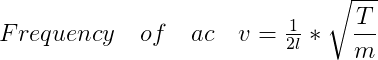Where T = Mg , the tension of the wire and 'm' is the linear density of the wire.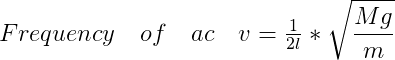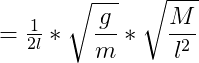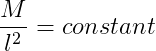If r is the radius of the wire, and  ρ is the density of its material, m = πr²ρ

The graph between mass (M) of the suspended weights and square of the resonating length (l2) by taking M along X-axis and l2 along Y-axis is drawn. The graph should be a straight sloping line. The slope of the line AB/BC gives the value of M/l2, which is a constant.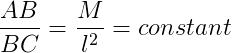# Learning Outcomes:

• Students will be able to relate the tension of the wire, linear density of the wire and the resonating   length of the wire.
• Students will be able to derive the relationship between frequency of alternating current and the resonating length of the wire.

Cite this Simulator: